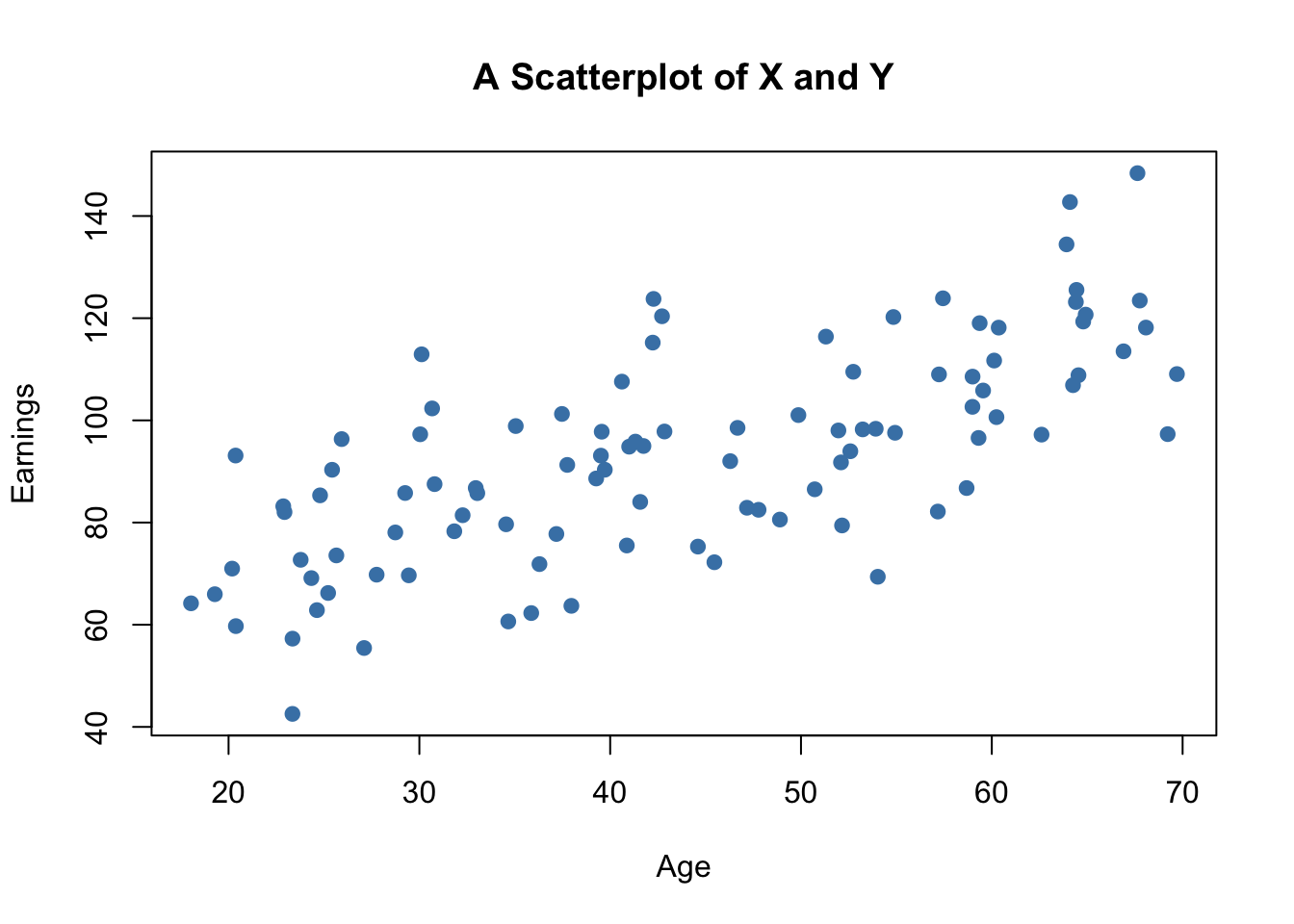This book is in Open Review. We want your feedback to make the book better for you and other students. You may annotate some text by selecting it with the cursor and then click the on the pop-up menu. You can also see the annotations of others: click the in the upper right hand corner of the page

3.7 Scatterplots, Sample Covariance and Sample Correlation

A scatter plot represents two dimensional data, for example $n$ observation on $X_i$ and $Y_i$, by points in a coordinate system. It is very easy to generate scatter plots using the plot() function in R. Let us generate some artificial data on age and earnings of workers and plot it.

# set random seed
set.seed(123)

# generate dataset
X <- runif(n = 100,
min = 18,
max = 70)

Y <- X + rnorm(n=100, 50, 15)

# plot observations
plot(X,
Y,
type = "p",
main = "A Scatterplot of X and Y",
xlab = "Age",
ylab = "Earnings",
col = "steelblue",
pch = 19)The plot shows positive correlation between age and earnings. This is in line with the notion that older workers earn more than those who joined the working population recently.

Sample Covariance and Correlation

By now you should be familiar with the concepts of variance and covariance. If not, we recommend you to work your way through Chapter 2 of the book.

Just like the variance, covariance and correlation of two variables are properties that relate to the (unknown) joint probability distribution of these variables. We can estimate covariance and correlation by means of suitable estimators using a sample $(X_i,Y_i)$, $i=1,\dots,n$.

The sample covariance

$s_{XY} = \frac{1}{n-1} \sum_{i=1}^n (X_i - \overline{X})(Y_i - \overline{Y})$

is an estimator for the population variance of $X$ and $Y$ whereas the sample correlation

$r_{XY} = \frac{s_{XY}}{s_Xs_Y}$ can be used to estimate the population correlation, a standardized measure for the strength of the linear relationship between $X$ and $Y$. See Chapter 3.7 in the book for a more detailed treatment of these estimators.

As for variance and standard deviation, these estimators are implemented as R functions in the stats package. We can use them to estimate population covariance and population correlation of the artificial data on age and earnings.

# compute sample covariance of X and Y
cov(X, Y)
#>  213.934

# compute sample correlation between X and Y
cor(X, Y)
#>  0.706372

# an equivalent way to compute the sample correlation
cov(X, Y) / (sd(X) * sd(Y))
#>  0.706372

The estimates indicate that $X$ and $Y$ are moderately correlated.

The next code chunk uses the function mvnorm() from package MASS to generate bivariate sample data with different degrees of correlation.

library(MASS)

# set random seed
set.seed(1)

# positive correlation (0.81)
example1 <- mvrnorm(100,
mu = c(0, 0),
Sigma = matrix(c(2, 2, 2, 3), ncol = 2),
empirical = TRUE)

# negative correlation (-0.81)
example2 <- mvrnorm(100,
mu = c(0, 0),
Sigma = matrix(c(2, -2, -2, 3), ncol = 2),
empirical = TRUE)

# no correlation
example3 <- mvrnorm(100,
mu = c(0, 0),
Sigma = matrix(c(1, 0, 0, 1), ncol = 2),
empirical = TRUE)

X <- seq(-3, 3, 0.01)
Y <- - X^2 + rnorm(length(X))

example4 <- cbind(X, Y)

# divide plot area as 2-by-2 array
par(mfrow = c(2, 2))

# plot datasets
plot(example1, col = "steelblue", pch = 20, xlab = "X", ylab = "Y",
main = "Correlation = 0.81")

plot(example2, col = "steelblue", pch = 20, xlab = "X", ylab = "Y",
main = "Correlation = -0.81")

plot(example3, col = "steelblue", pch = 20, xlab = "X", ylab = "Y",
main = "Correlation = 0")

plot(example4, col = "steelblue", pch = 20, xlab = "X", ylab = "Y",
main = "Correlation = 0")References

Ripley, Brian. 2021. MASS: Support Functions and Datasets for Venables and Ripley’s MASS (version 7.3-54). http://www.stats.ox.ac.uk/pub/MASS4/.